Скачать презентацию FINC 3131 Business Finance Chapter 12 Cash Flow

df59e38f9ff5981e6854cb922812008b.ppt

• Количество слайдов: 50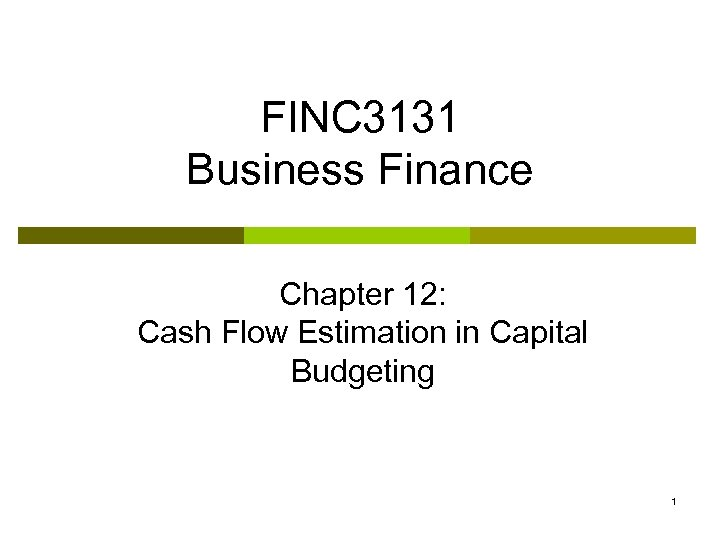FINC 3131 Business Finance Chapter 12: Cash Flow Estimation in Capital Budgeting 1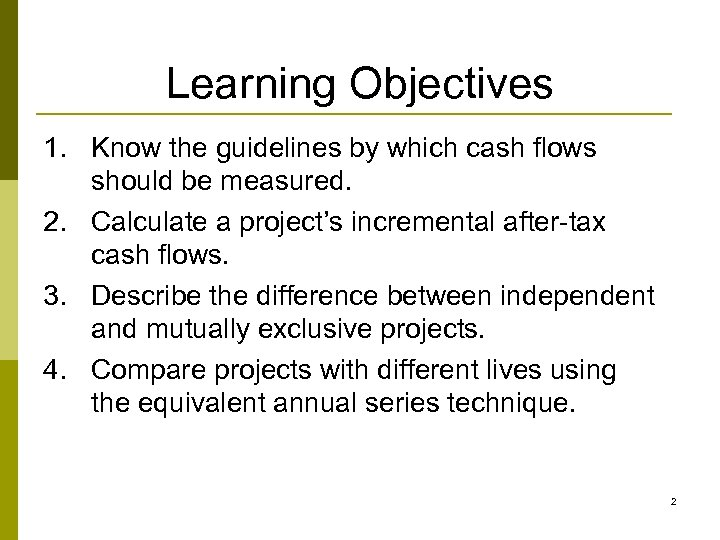Learning Objectives 1. Know the guidelines by which cash flows should be measured. 2. Calculate a project’s incremental after-tax cash flows. 3. Describe the difference between independent and mutually exclusive projects. 4. Compare projects with different lives using the equivalent annual series technique. 2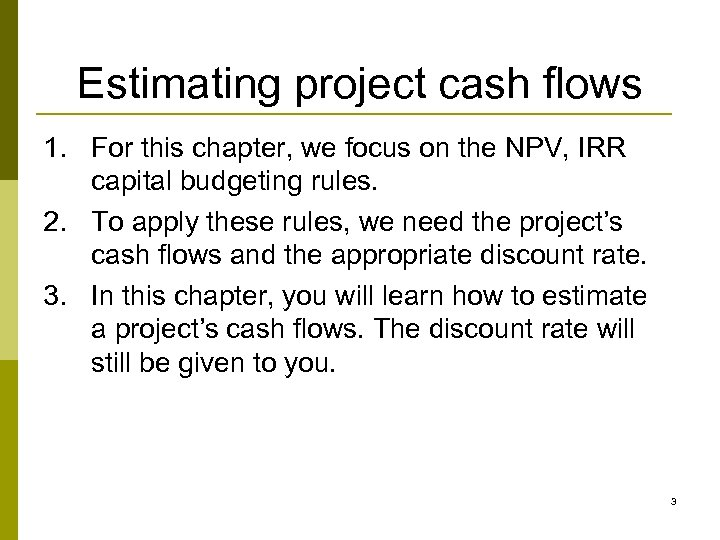Estimating project cash flows 1. For this chapter, we focus on the NPV, IRR capital budgeting rules. 2. To apply these rules, we need the project’s cash flows and the appropriate discount rate. 3. In this chapter, you will learn how to estimate a project’s cash flows. The discount rate will still be given to you. 3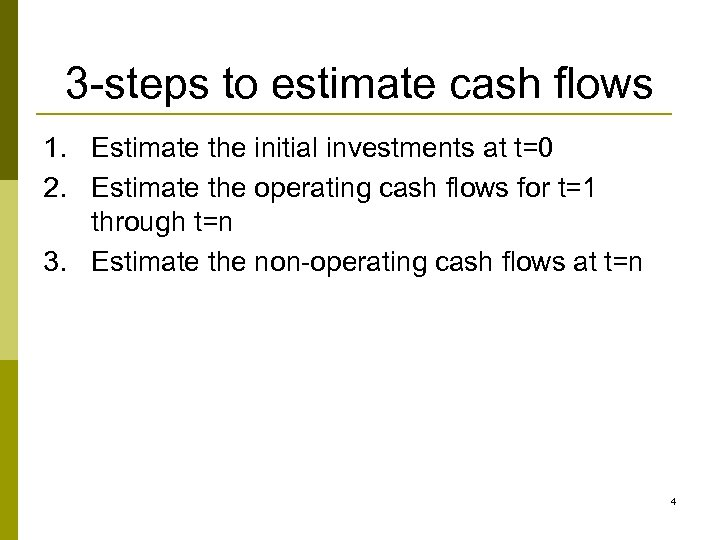3 -steps to estimate cash flows 1. Estimate the initial investments at t=0 2. Estimate the operating cash flows for t=1 through t=n 3. Estimate the non-operating cash flows at t=n 4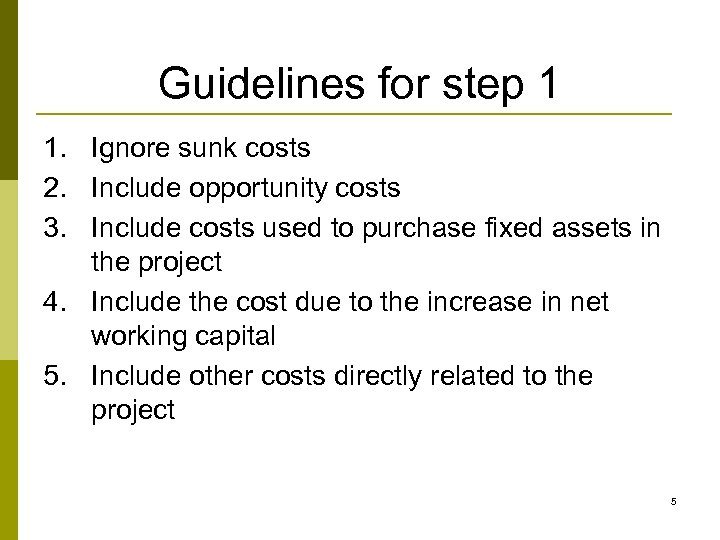Guidelines for step 1 1. Ignore sunk costs 2. Include opportunity costs 3. Include costs used to purchase fixed assets in the project 4. Include the cost due to the increase in net working capital 5. Include other costs directly related to the project 5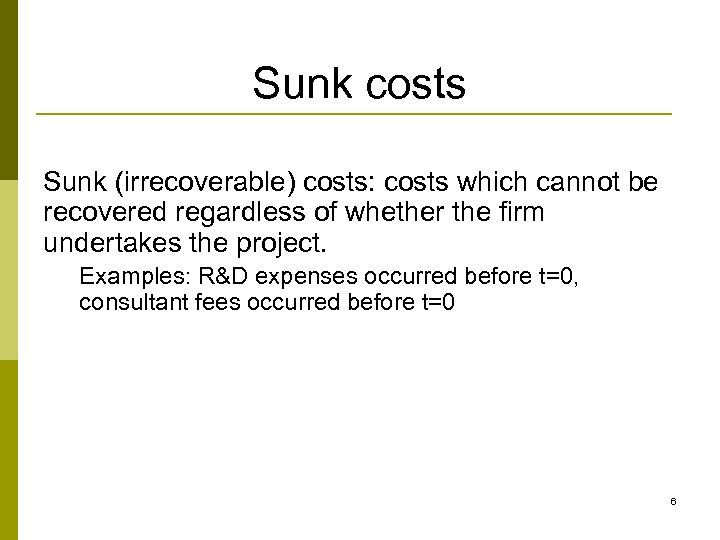Sunk costs Sunk (irrecoverable) costs: costs which cannot be recovered regardless of whether the firm undertakes the project. Examples: R&D expenses occurred before t=0, consultant fees occurred before t=0 6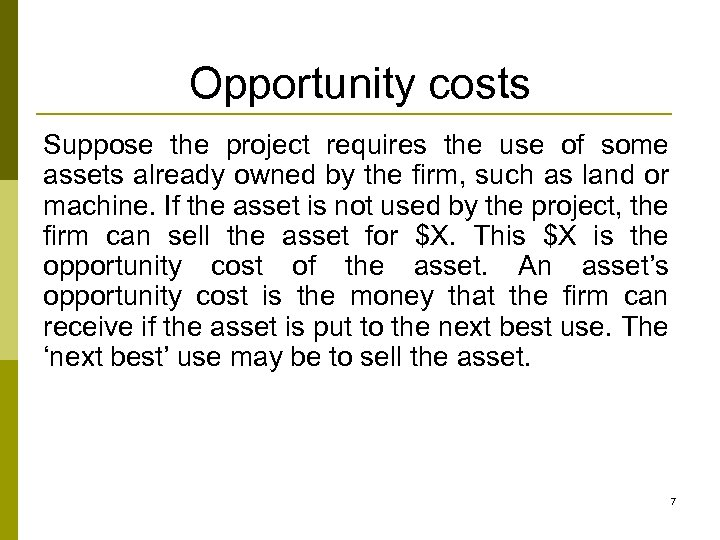Opportunity costs Suppose the project requires the use of some assets already owned by the firm, such as land or machine. If the asset is not used by the project, the firm can sell the asset for \$X. This \$X is the opportunity cost of the asset. An asset’s opportunity cost is the money that the firm can receive if the asset is put to the next best use. The ‘next best’ use may be to sell the asset. 7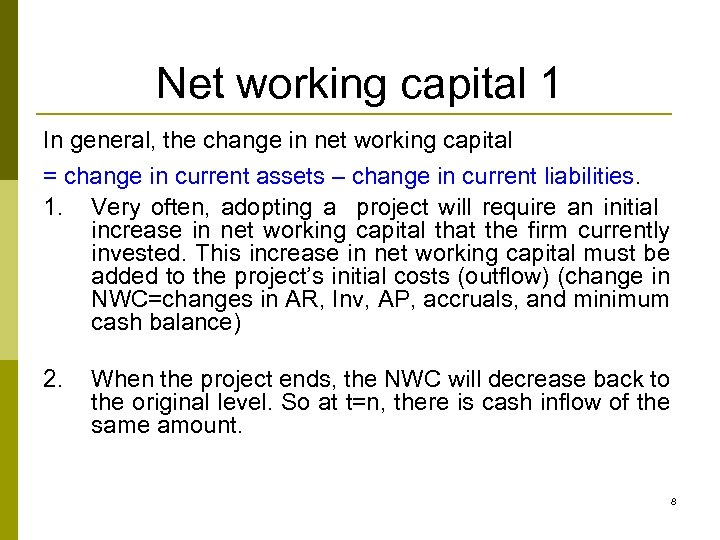Net working capital 1 In general, the change in net working capital = change in current assets – change in current liabilities. 1. Very often, adopting a project will require an initial increase in net working capital that the firm currently invested. This increase in net working capital must be added to the project’s initial costs (outflow) (change in NWC=changes in AR, Inv, AP, accruals, and minimum cash balance) 2. When the project ends, the NWC will decrease back to the original level. So at t=n, there is cash inflow of the same amount. 8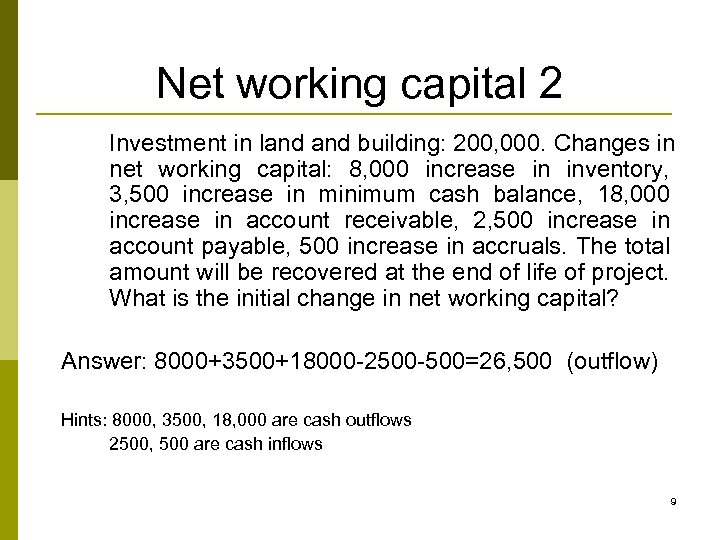Net working capital 2 Investment in land building: 200, 000. Changes in net working capital: 8, 000 increase in inventory, 3, 500 increase in minimum cash balance, 18, 000 increase in account receivable, 2, 500 increase in account payable, 500 increase in accruals. The total amount will be recovered at the end of life of project. What is the initial change in net working capital? Answer: 8000+3500+18000 -2500 -500=26, 500 (outflow) Hints: 8000, 3500, 18, 000 are cash outflows 2500, 500 are cash inflows 9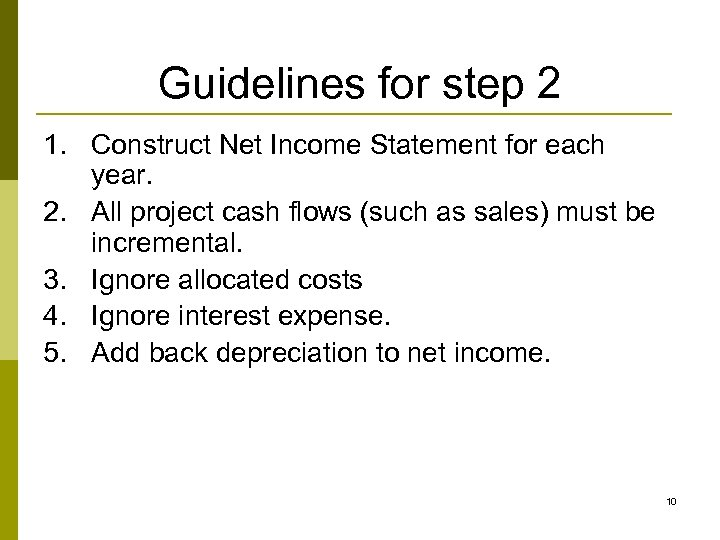Guidelines for step 2 1. Construct Net Income Statement for each year. 2. All project cash flows (such as sales) must be incremental. 3. Ignore allocated costs 4. Ignore interest expense. 5. Add back depreciation to net income. 10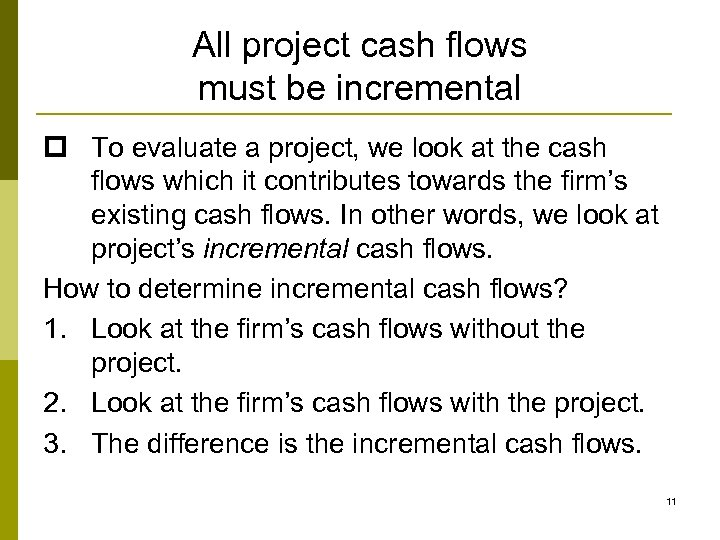All project cash flows must be incremental p To evaluate a project, we look at the cash flows which it contributes towards the firm’s existing cash flows. In other words, we look at project’s incremental cash flows. How to determine incremental cash flows? 1. Look at the firm’s cash flows without the project. 2. Look at the firm’s cash flows with the project. 3. The difference is the incremental cash flows. 11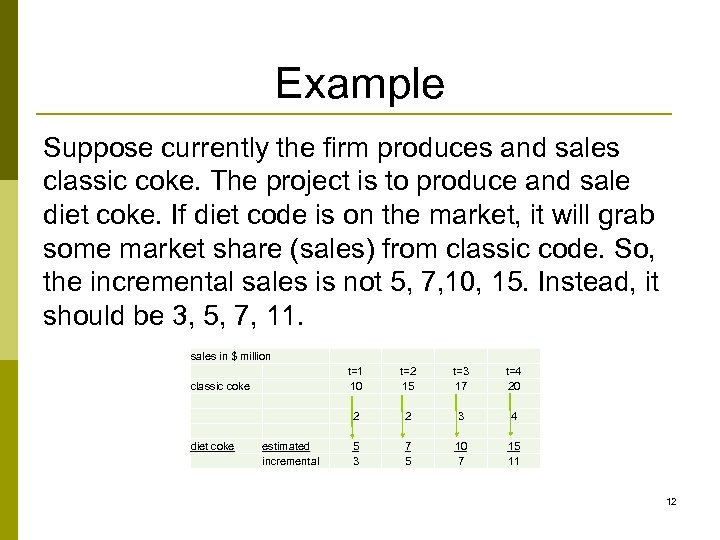Example Suppose currently the firm produces and sales classic coke. The project is to produce and sale diet coke. If diet code is on the market, it will grab some market share (sales) from classic code. So, the incremental sales is not 5, 7, 10, 15. Instead, it should be 3, 5, 7, 11. sales in \$ million diet coke estimated incremental t=2 15 t=3 17 t=4 20 2 classic coke t=1 10 2 3 4 5 3 7 5 10 7 15 11 12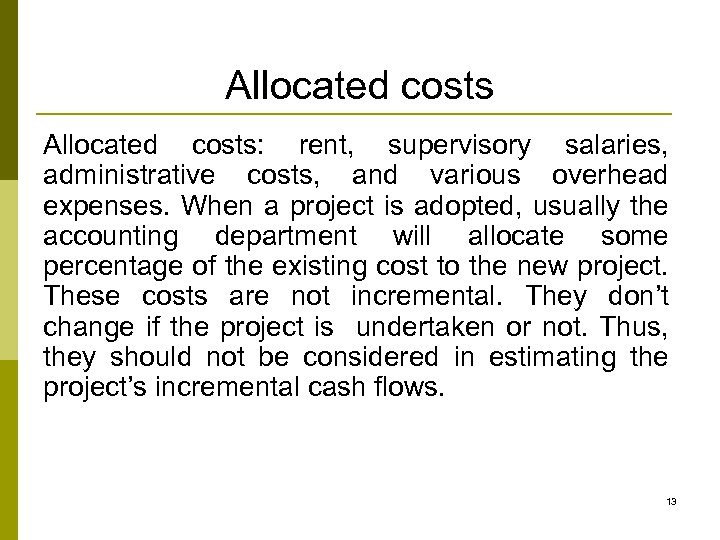Allocated costs: rent, supervisory salaries, administrative costs, and various overhead expenses. When a project is adopted, usually the accounting department will allocate some percentage of the existing cost to the new project. These costs are not incremental. They don’t change if the project is undertaken or not. Thus, they should not be considered in estimating the project’s incremental cash flows. 13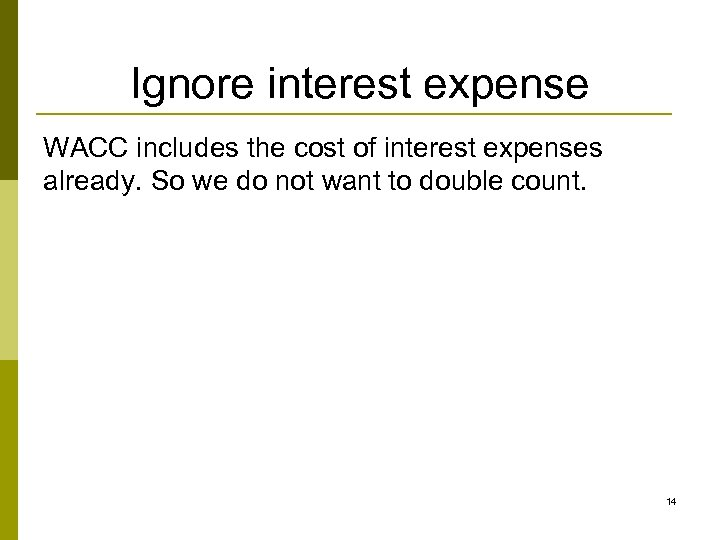Ignore interest expense WACC includes the cost of interest expenses already. So we do not want to double count. 14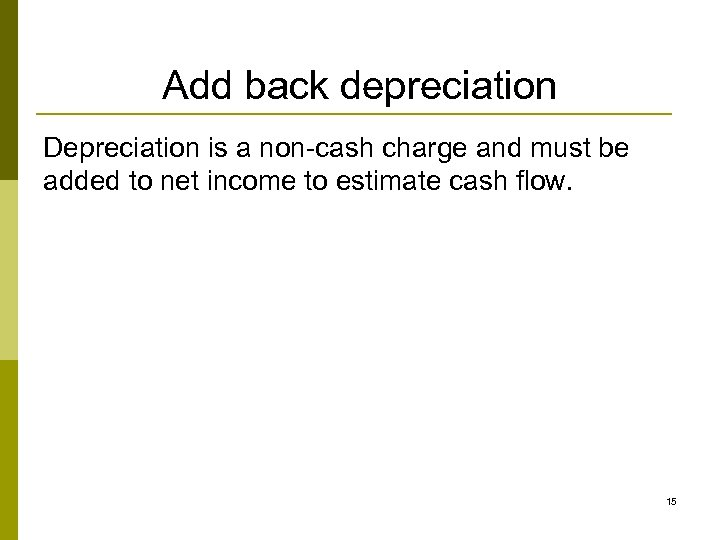Add back depreciation Depreciation is a non-cash charge and must be added to net income to estimate cash flow. 15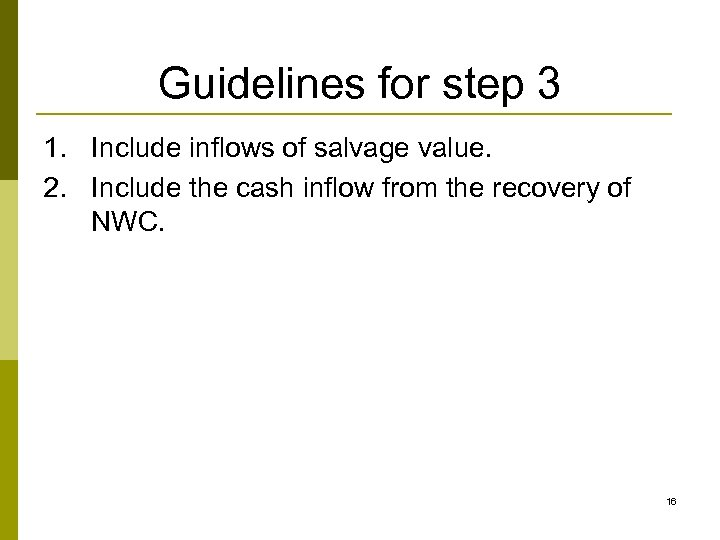Guidelines for step 3 1. Include inflows of salvage value. 2. Include the cash inflow from the recovery of NWC. 16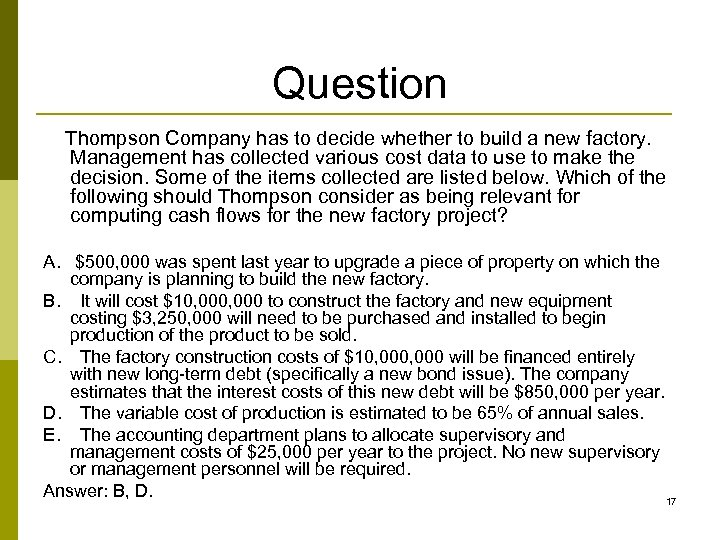Question Thompson Company has to decide whether to build a new factory. Management has collected various cost data to use to make the decision. Some of the items collected are listed below. Which of the following should Thompson consider as being relevant for computing cash flows for the new factory project? A. \$500, 000 was spent last year to upgrade a piece of property on which the company is planning to build the new factory. B. It will cost \$10, 000 to construct the factory and new equipment costing \$3, 250, 000 will need to be purchased and installed to begin production of the product to be sold. C. The factory construction costs of \$10, 000 will be financed entirely with new long-term debt (specifically a new bond issue). The company estimates that the interest costs of this new debt will be \$850, 000 per year. D. The variable cost of production is estimated to be 65% of annual sales. E. The accounting department plans to allocate supervisory and management costs of \$25, 000 per year to the project. No new supervisory or management personnel will be required. Answer: B, D. 17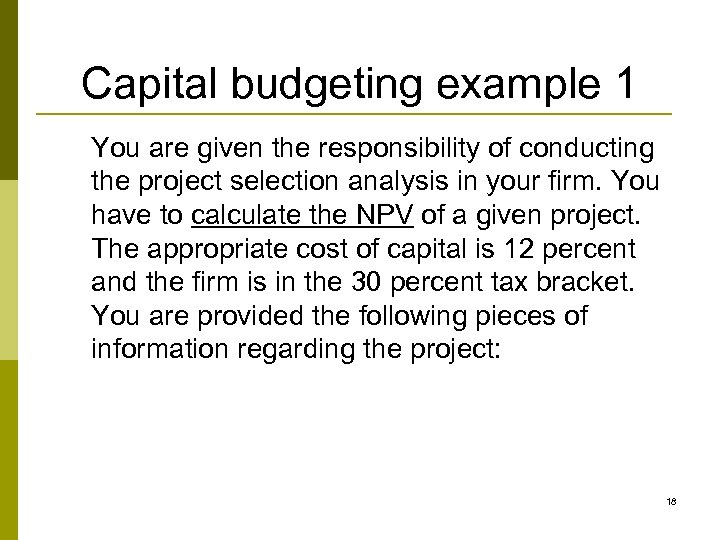Capital budgeting example 1 You are given the responsibility of conducting the project selection analysis in your firm. You have to calculate the NPV of a given project. The appropriate cost of capital is 12 percent and the firm is in the 30 percent tax bracket. You are provided the following pieces of information regarding the project: 18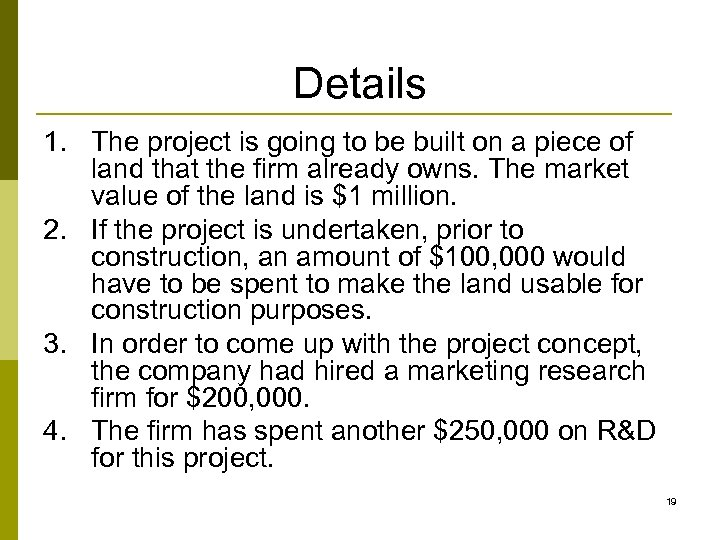Details 1. The project is going to be built on a piece of land that the firm already owns. The market value of the land is \$1 million. 2. If the project is undertaken, prior to construction, an amount of \$100, 000 would have to be spent to make the land usable for construction purposes. 3. In order to come up with the project concept, the company had hired a marketing research firm for \$200, 000. 4. The firm has spent another \$250, 000 on R&D for this project. 19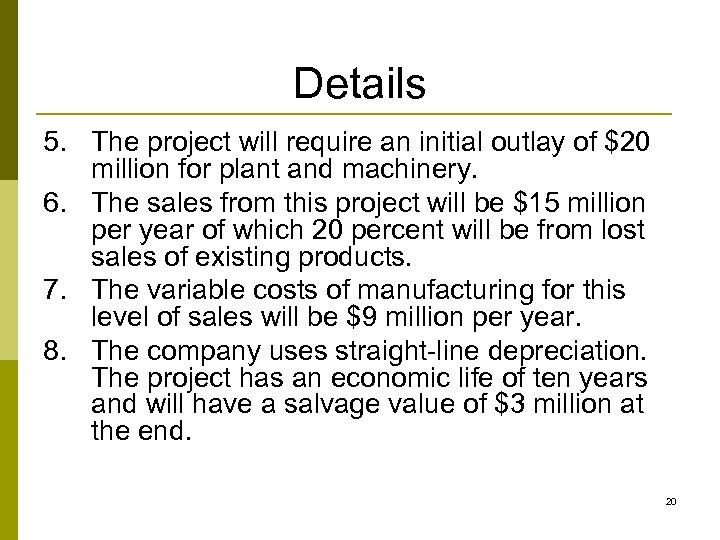Details 5. The project will require an initial outlay of \$20 million for plant and machinery. 6. The sales from this project will be \$15 million per year of which 20 percent will be from lost sales of existing products. 7. The variable costs of manufacturing for this level of sales will be \$9 million per year. 8. The company uses straight-line depreciation. The project has an economic life of ten years and will have a salvage value of \$3 million at the end. 20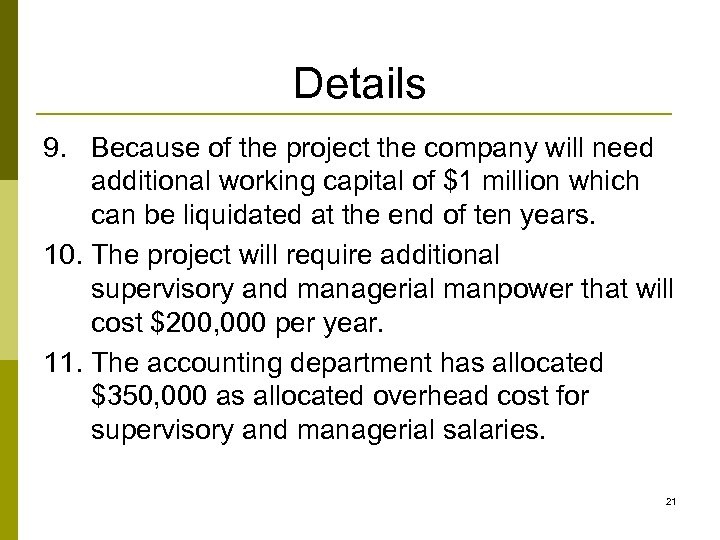Details 9. Because of the project the company will need additional working capital of \$1 million which can be liquidated at the end of ten years. 10. The project will require additional supervisory and managerial manpower that will cost \$200, 000 per year. 11. The accounting department has allocated \$350, 000 as allocated overhead cost for supervisory and managerial salaries. 21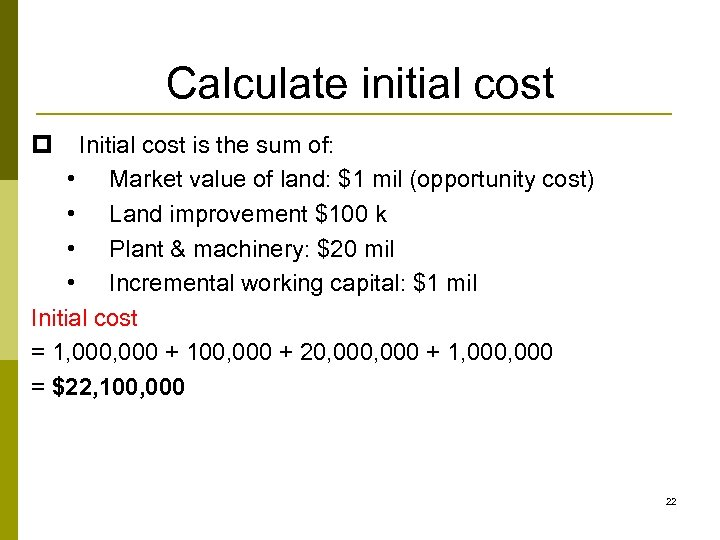Calculate initial cost p Initial cost is the sum of: • Market value of land: \$1 mil (opportunity cost) • Land improvement \$100 k • Plant & machinery: \$20 mil • Incremental working capital: \$1 mil Initial cost = 1, 000 + 100, 000 + 20, 000 + 1, 000 = \$22, 100, 000 22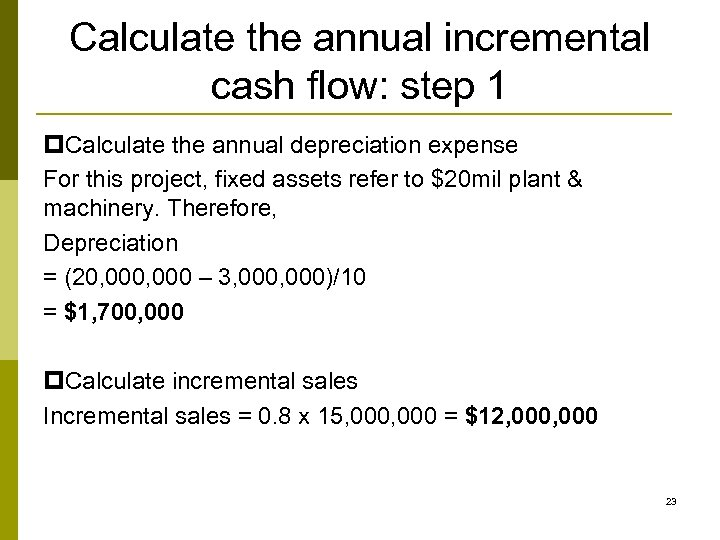Calculate the annual incremental cash flow: step 1 p. Calculate the annual depreciation expense For this project, fixed assets refer to \$20 mil plant & machinery. Therefore, Depreciation = (20, 000 – 3, 000)/10 = \$1, 700, 000 p. Calculate incremental sales Incremental sales = 0. 8 x 15, 000 = \$12, 000 23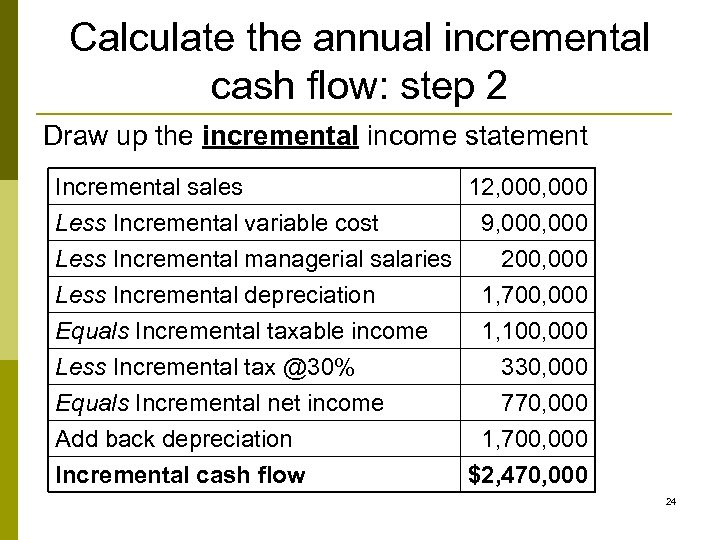Calculate the annual incremental cash flow: step 2 Draw up the incremental income statement Incremental sales 12, 000 Less Incremental variable cost 9, 000 Less Incremental managerial salaries 200, 000 Less Incremental depreciation 1, 700, 000 Equals Incremental taxable income Less Incremental tax @30% Equals Incremental net income Add back depreciation Incremental cash flow 1, 100, 000 330, 000 770, 000 1, 700, 000 \$2, 470, 000 24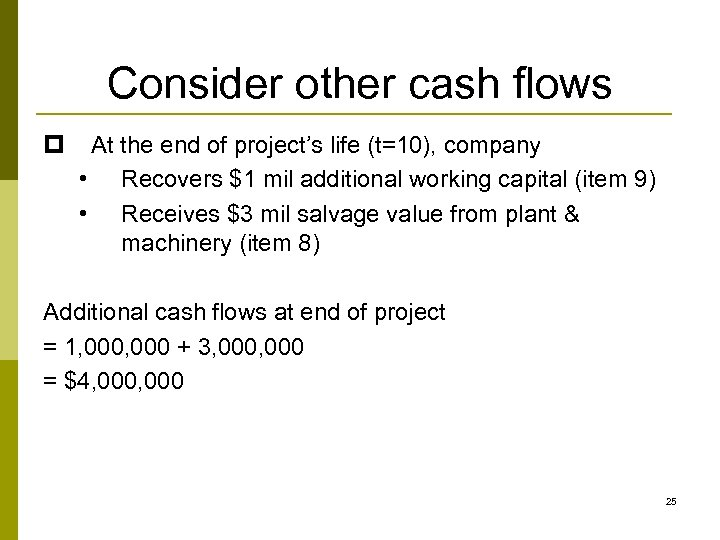Consider other cash flows p At the end of project’s life (t=10), company • Recovers \$1 mil additional working capital (item 9) • Receives \$3 mil salvage value from plant & machinery (item 8) Additional cash flows at end of project = 1, 000 + 3, 000 = \$4, 000 25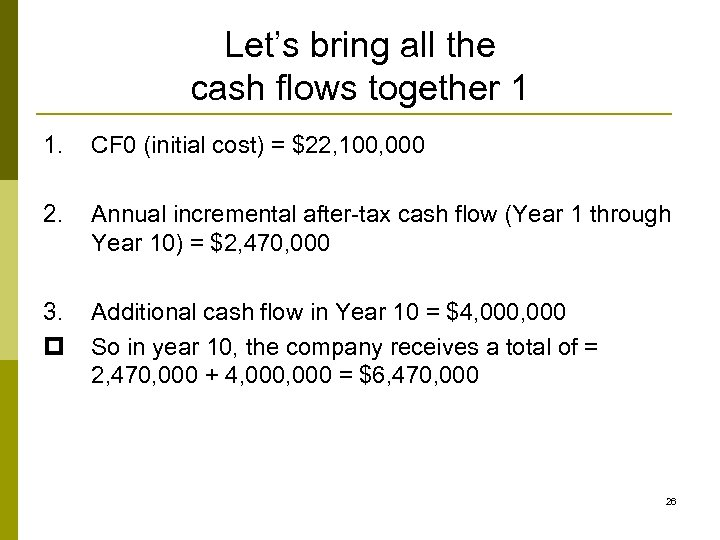Let’s bring all the cash flows together 1 1. CF 0 (initial cost) = \$22, 100, 000 2. Annual incremental after-tax cash flow (Year 1 through Year 10) = \$2, 470, 000 3. p Additional cash flow in Year 10 = \$4, 000 So in year 10, the company receives a total of = 2, 470, 000 + 4, 000 = \$6, 470, 000 26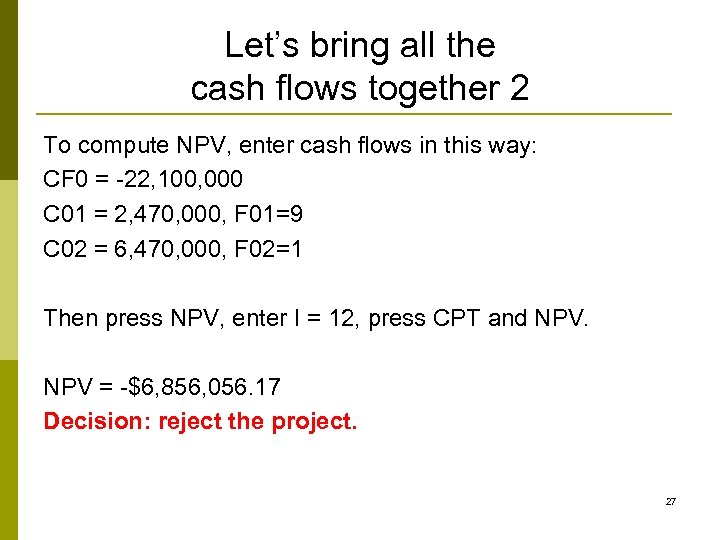Let’s bring all the cash flows together 2 To compute NPV, enter cash flows in this way: CF 0 = -22, 100, 000 C 01 = 2, 470, 000, F 01=9 C 02 = 6, 470, 000, F 02=1 Then press NPV, enter I = 12, press CPT and NPV = -\$6, 856, 056. 17 Decision: reject the project. 27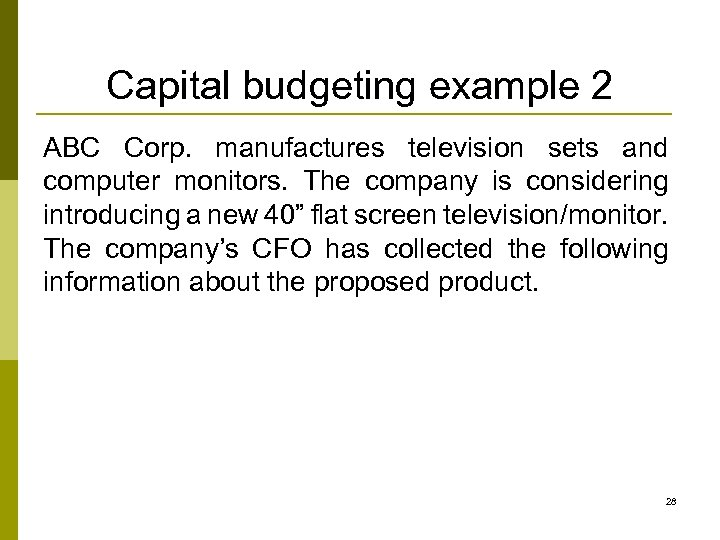Capital budgeting example 2 ABC Corp. manufactures television sets and computer monitors. The company is considering introducing a new 40” flat screen television/monitor. The company’s CFO has collected the following information about the proposed product. 28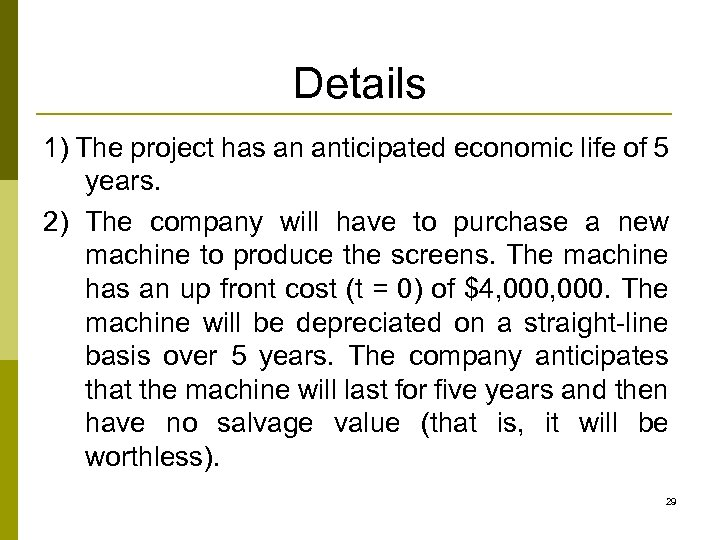Details 1) The project has an anticipated economic life of 5 years. 2) The company will have to purchase a new machine to produce the screens. The machine has an up front cost (t = 0) of \$4, 000. The machine will be depreciated on a straight-line basis over 5 years. The company anticipates that the machine will last for five years and then have no salvage value (that is, it will be worthless). 29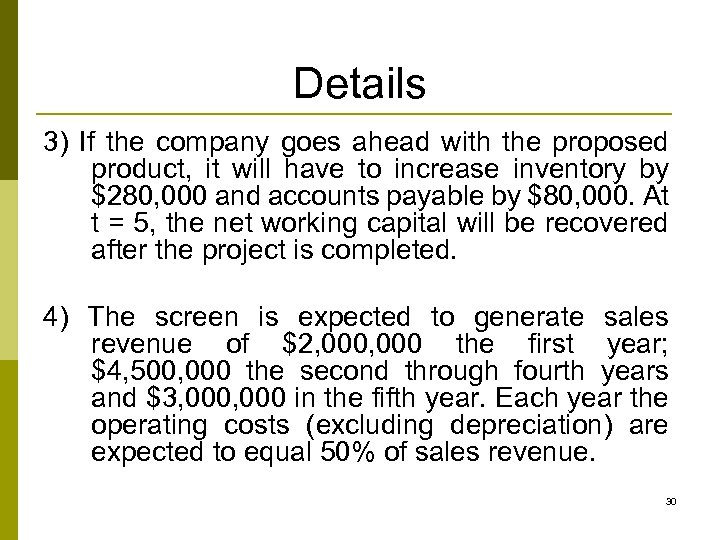Details 3) If the company goes ahead with the proposed product, it will have to increase inventory by \$280, 000 and accounts payable by \$80, 000. At t = 5, the net working capital will be recovered after the project is completed. 4) The screen is expected to generate sales revenue of \$2, 000 the first year; \$4, 500, 000 the second through fourth years and \$3, 000 in the fifth year. Each year the operating costs (excluding depreciation) are expected to equal 50% of sales revenue. 30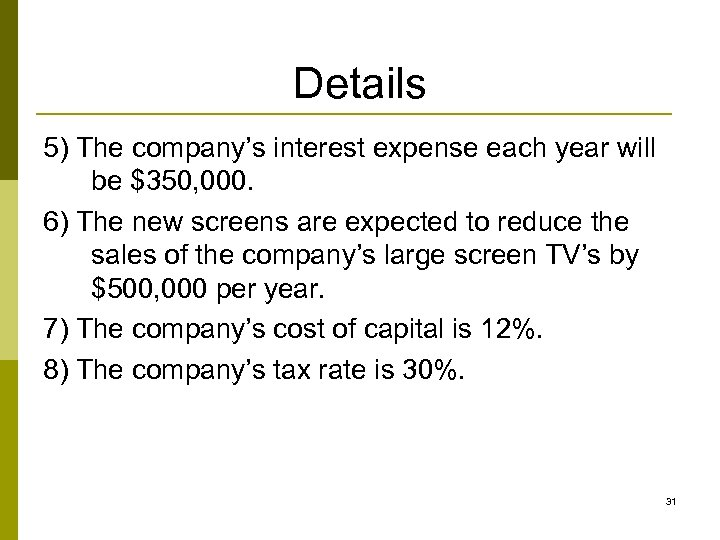Details 5) The company’s interest expense each year will be \$350, 000. 6) The new screens are expected to reduce the sales of the company’s large screen TV’s by \$500, 000 per year. 7) The company’s cost of capital is 12%. 8) The company’s tax rate is 30%. 31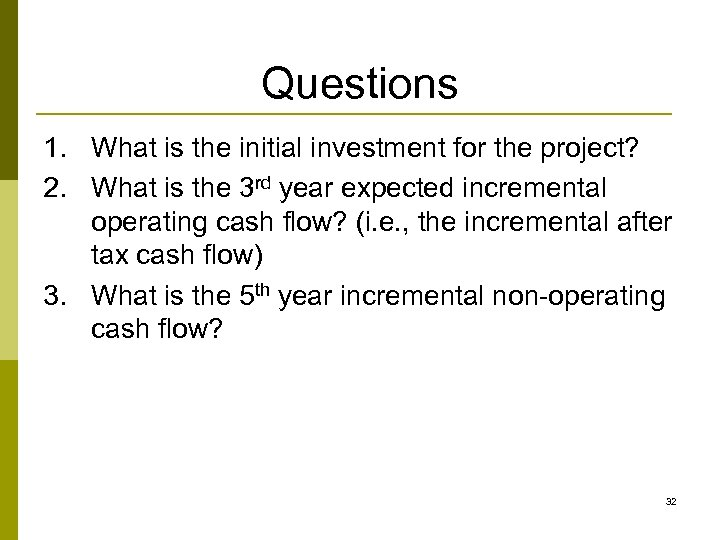Questions 1. What is the initial investment for the project? 2. What is the 3 rd year expected incremental operating cash flow? (i. e. , the incremental after tax cash flow) 3. What is the 5 th year incremental non-operating cash flow? 32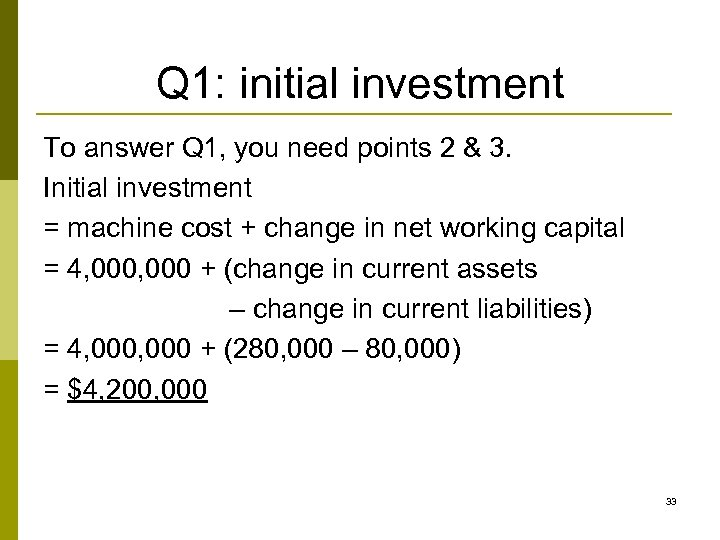Q 1: initial investment To answer Q 1, you need points 2 & 3. Initial investment = machine cost + change in net working capital = 4, 000 + (change in current assets – change in current liabilities) = 4, 000 + (280, 000 – 80, 000) = \$4, 200, 000 33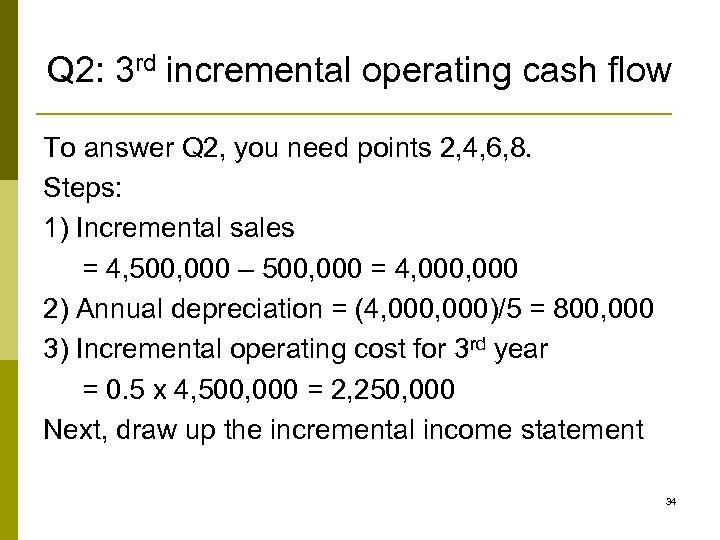Q 2: 3 rd incremental operating cash flow To answer Q 2, you need points 2, 4, 6, 8. Steps: 1) Incremental sales = 4, 500, 000 – 500, 000 = 4, 000 2) Annual depreciation = (4, 000)/5 = 800, 000 3) Incremental operating cost for 3 rd year = 0. 5 x 4, 500, 000 = 2, 250, 000 Next, draw up the incremental income statement 34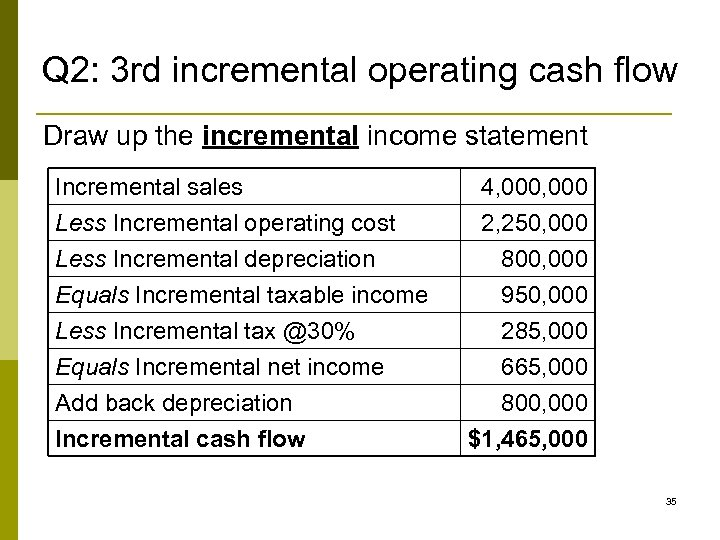Q 2: 3 rd incremental operating cash flow Draw up the incremental income statement Incremental sales Less Incremental operating cost Less Incremental depreciation Equals Incremental taxable income Less Incremental tax @30% Equals Incremental net income Add back depreciation Incremental cash flow 4, 000 2, 250, 000 800, 000 950, 000 285, 000 665, 000 800, 000 \$1, 465, 000 35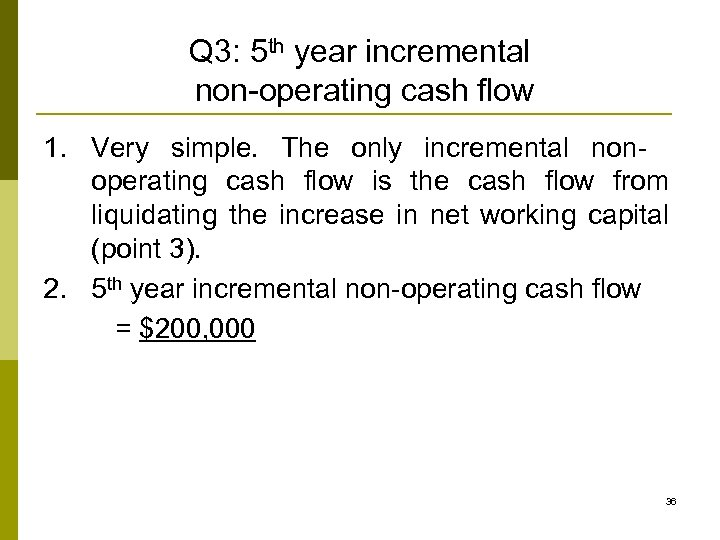Q 3: 5 th year incremental non-operating cash flow 1. Very simple. The only incremental nonoperating cash flow is the cash flow from liquidating the increase in net working capital (point 3). 2. 5 th year incremental non-operating cash flow = \$200, 000 36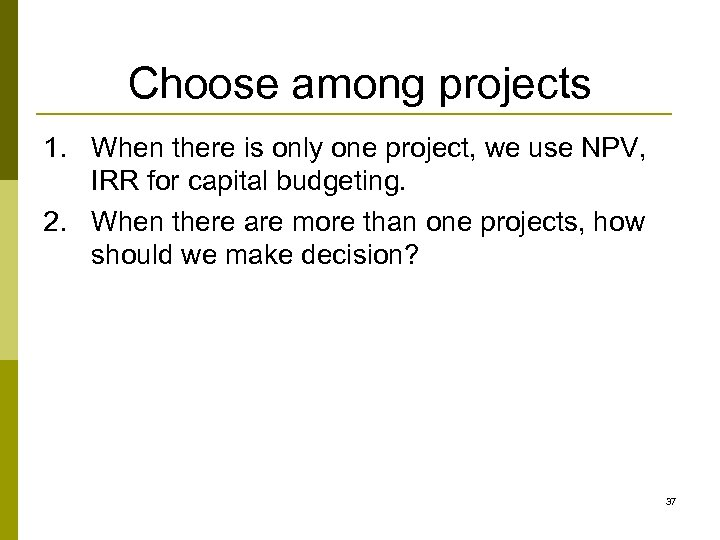Choose among projects 1. When there is only one project, we use NPV, IRR for capital budgeting. 2. When there are more than one projects, how should we make decision? 37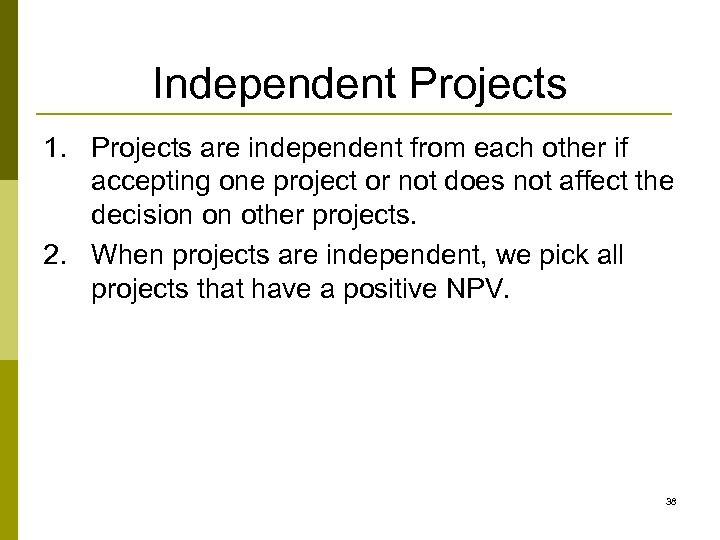Independent Projects 1. Projects are independent from each other if accepting one project or not does not affect the decision on other projects. 2. When projects are independent, we pick all projects that have a positive NPV. 38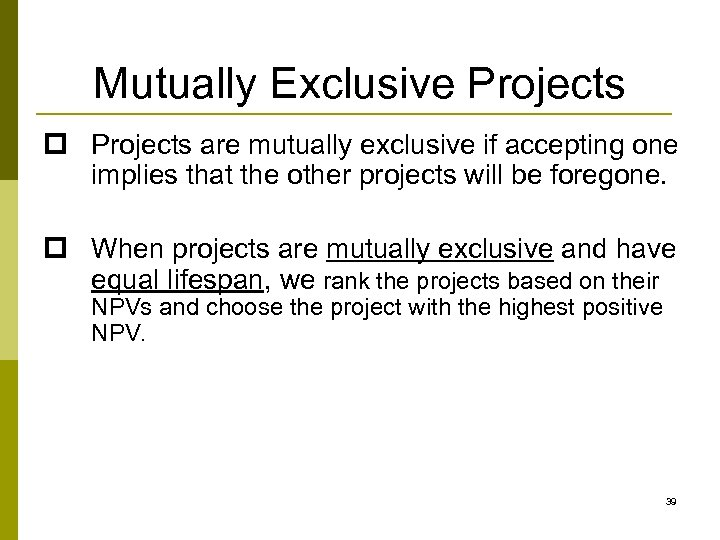Mutually Exclusive Projects p Projects are mutually exclusive if accepting one implies that the other projects will be foregone. p When projects are mutually exclusive and have equal lifespan, we rank the projects based on their NPVs and choose the project with the highest positive NPV. 39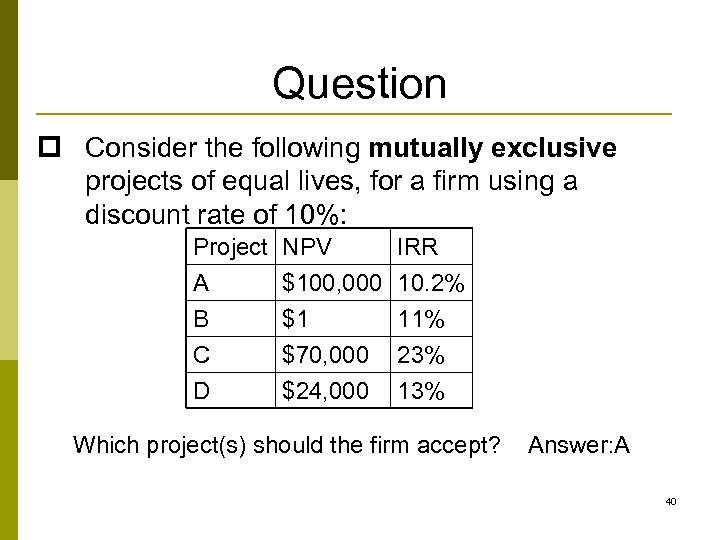Question p Consider the following mutually exclusive projects of equal lives, for a firm using a discount rate of 10%: Project A B C NPV \$100, 000 \$1 \$70, 000 IRR 10. 2% 11% 23% D \$24, 000 13% Which project(s) should the firm accept? Answer: A 40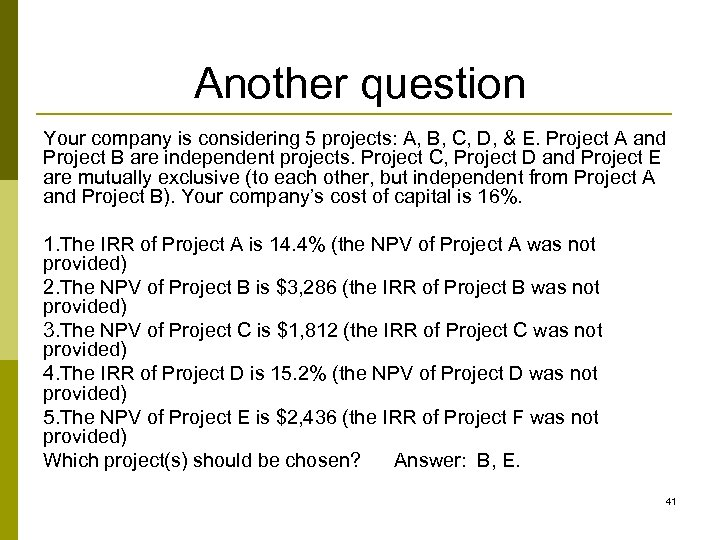Another question Your company is considering 5 projects: A, B, C, D, & E. Project A and Project B are independent projects. Project C, Project D and Project E are mutually exclusive (to each other, but independent from Project A and Project B). Your company’s cost of capital is 16%. 1. The IRR of Project A is 14. 4% (the NPV of Project A was not provided) 2. The NPV of Project B is \$3, 286 (the IRR of Project B was not provided) 3. The NPV of Project C is \$1, 812 (the IRR of Project C was not provided) 4. The IRR of Project D is 15. 2% (the NPV of Project D was not provided) 5. The NPV of Project E is \$2, 436 (the IRR of Project F was not provided) Which project(s) should be chosen? Answer: B, E. 41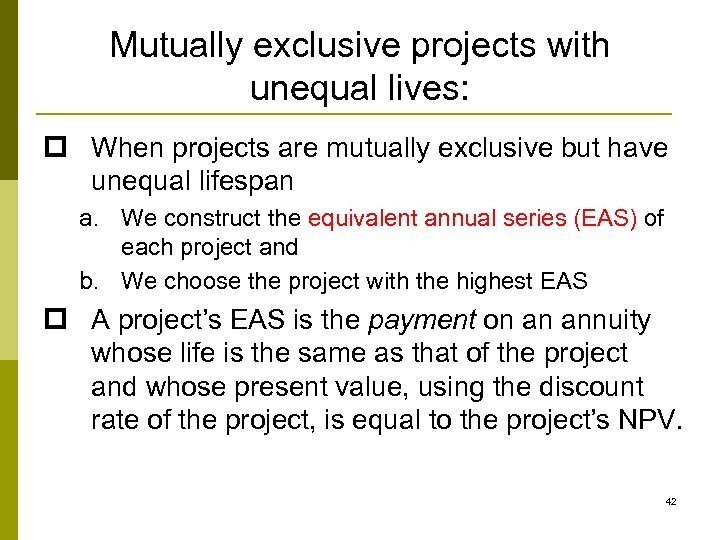Mutually exclusive projects with unequal lives: p When projects are mutually exclusive but have unequal lifespan a. We construct the equivalent annual series (EAS) of each project and b. We choose the project with the highest EAS p A project’s EAS is the payment on an annuity whose life is the same as that of the project and whose present value, using the discount rate of the project, is equal to the project’s NPV. 42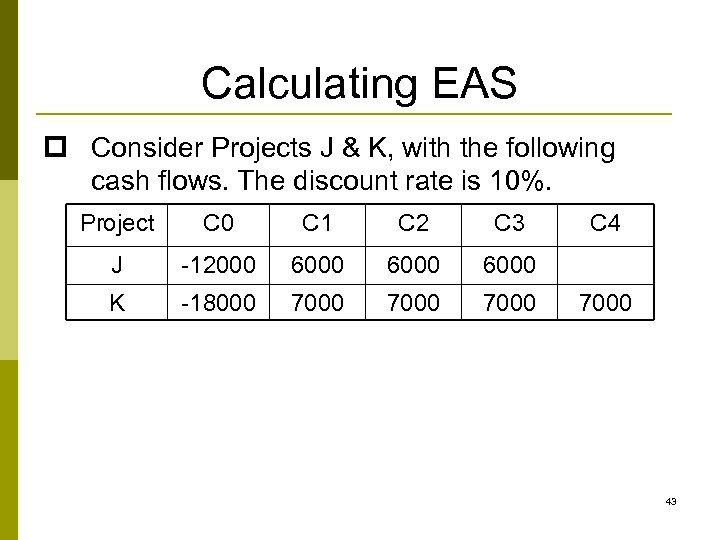Calculating EAS p Consider Projects J & K, with the following cash flows. The discount rate is 10%. Project C 0 C 1 C 2 C 3 J -12000 6000 K -18000 7000 C 4 7000 43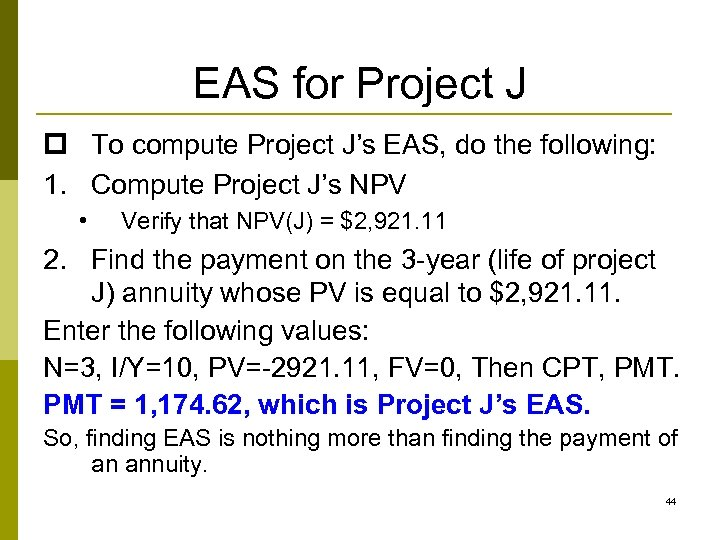EAS for Project J p To compute Project J’s EAS, do the following: 1. Compute Project J’s NPV • Verify that NPV(J) = \$2, 921. 11 2. Find the payment on the 3 -year (life of project J) annuity whose PV is equal to \$2, 921. 11. Enter the following values: N=3, I/Y=10, PV=-2921. 11, FV=0, Then CPT, PMT = 1, 174. 62, which is Project J’s EAS. So, finding EAS is nothing more than finding the payment of an annuity. 44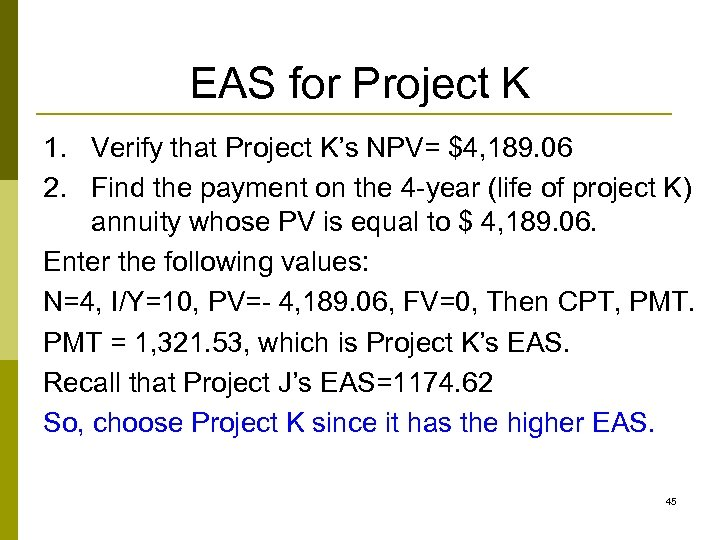EAS for Project K 1. Verify that Project K’s NPV= \$4, 189. 06 2. Find the payment on the 4 -year (life of project K) annuity whose PV is equal to \$ 4, 189. 06. Enter the following values: N=4, I/Y=10, PV=- 4, 189. 06, FV=0, Then CPT, PMT = 1, 321. 53, which is Project K’s EAS. Recall that Project J’s EAS=1174. 62 So, choose Project K since it has the higher EAS. 45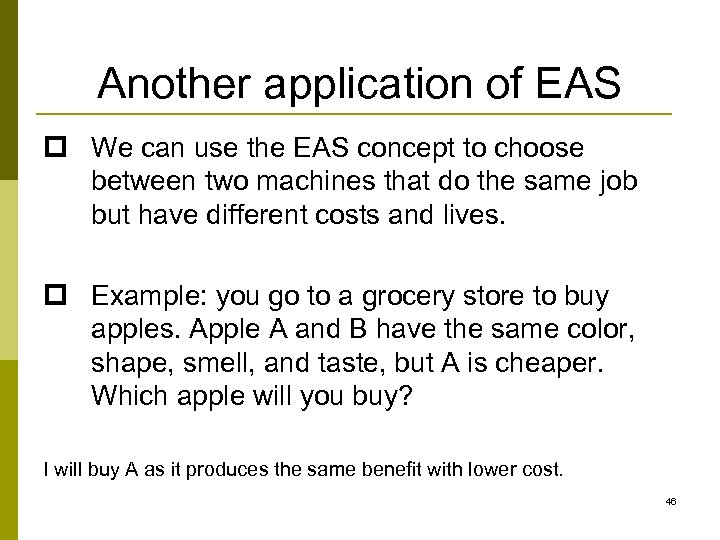Another application of EAS p We can use the EAS concept to choose between two machines that do the same job but have different costs and lives. p Example: you go to a grocery store to buy apples. Apple A and B have the same color, shape, smell, and taste, but A is cheaper. Which apple will you buy? I will buy A as it produces the same benefit with lower cost. 46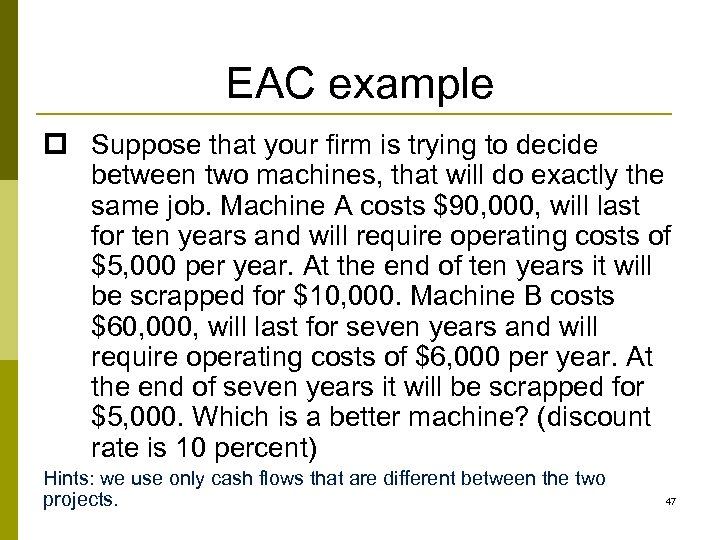EAC example p Suppose that your firm is trying to decide between two machines, that will do exactly the same job. Machine A costs \$90, 000, will last for ten years and will require operating costs of \$5, 000 per year. At the end of ten years it will be scrapped for \$10, 000. Machine B costs \$60, 000, will last for seven years and will require operating costs of \$6, 000 per year. At the end of seven years it will be scrapped for \$5, 000. Which is a better machine? (discount rate is 10 percent) Hints: we use only cash flows that are different between the two projects. 47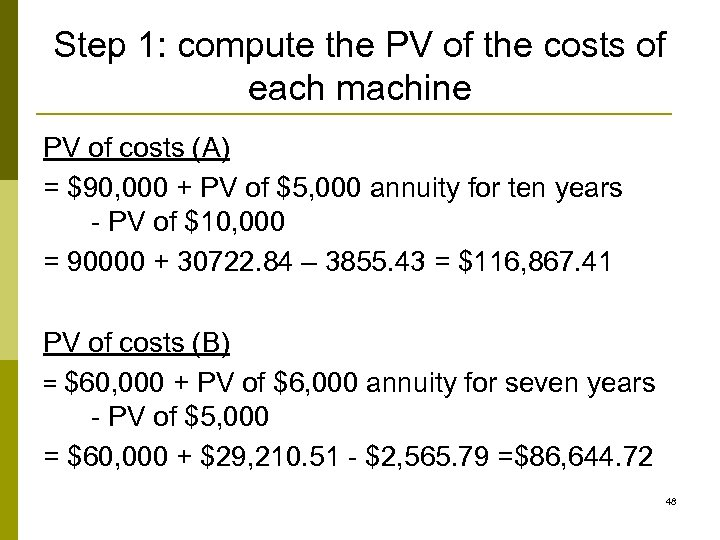Step 1: compute the PV of the costs of each machine PV of costs (A) = \$90, 000 + PV of \$5, 000 annuity for ten years - PV of \$10, 000 = 90000 + 30722. 84 – 3855. 43 = \$116, 867. 41 PV of costs (B) = \$60, 000 + PV of \$6, 000 annuity for seven years - PV of \$5, 000 = \$60, 000 + \$29, 210. 51 - \$2, 565. 79 =\$86, 644. 72 48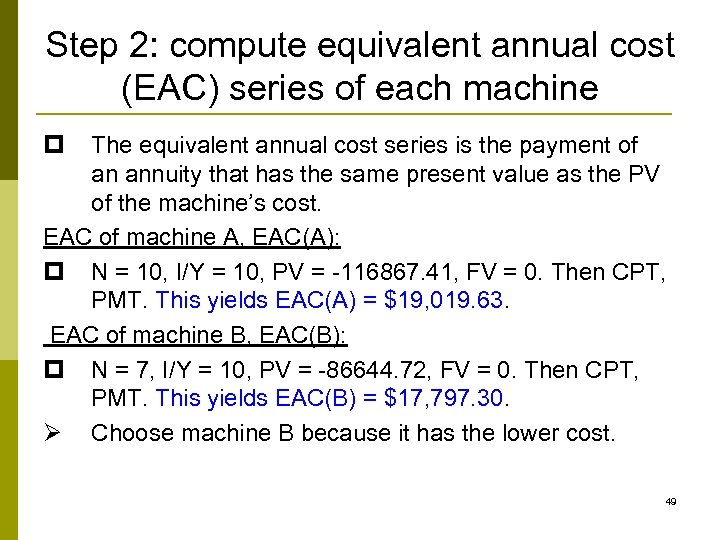Step 2: compute equivalent annual cost (EAC) series of each machine p The equivalent annual cost series is the payment of an annuity that has the same present value as the PV of the machine’s cost. EAC of machine A, EAC(A): p N = 10, I/Y = 10, PV = -116867. 41, FV = 0. Then CPT, PMT. This yields EAC(A) = \$19, 019. 63. EAC of machine B, EAC(B): p N = 7, I/Y = 10, PV = -86644. 72, FV = 0. Then CPT, PMT. This yields EAC(B) = \$17, 797. 30. Ø Choose machine B because it has the lower cost. 49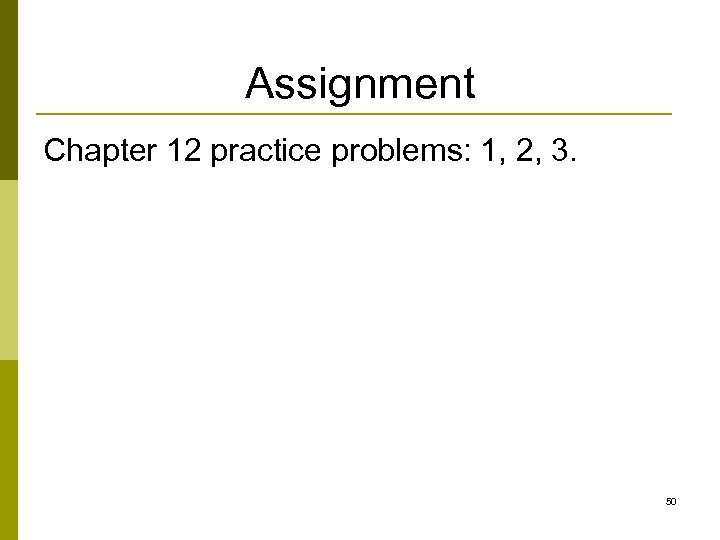Assignment Chapter 12 practice problems: 1, 2, 3. 50